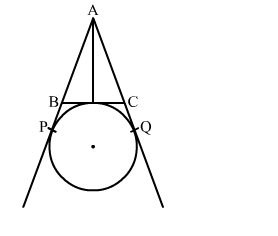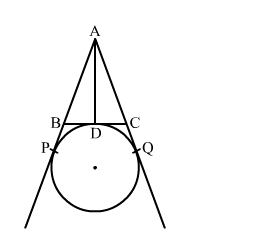# In the given figure, AP, AQ and BC are tangents to the circle.`
Question:

In the given figure, AP, AQ and BC are tangents to the circle. If AB = 5 cm, AC = 6 cm and BC = 4 cm then the length of AP is

(a) 15 cm
(b) 10 cm
(c) 9 cm
(d) 7.5 cmSolution:We know that tangent segments to a circle from the same external point are congruent.
Therefore, we have
AP = AQ
BP = BD
CQ = CD
Now, AB + BC + AC = 5 + 4 + 6 = 15
⇒AB + BD + DC + AC = 15 cm
⇒AB + BP + CQ + AC = 15 cm
⇒AP + AQ= 15 cm
⇒2AP = 15 cm
⇒AP = 7.5 cm
Hence, the correct answer is option(d)